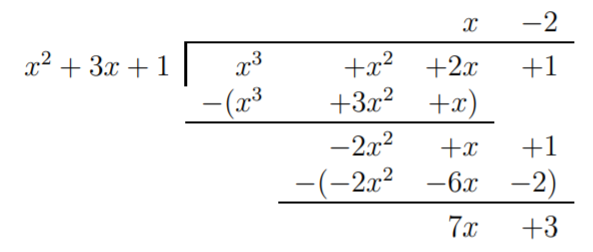# 8.1: Long division

$$\newcommand{\vecs}{\overset { \rightharpoonup} {\mathbf{#1}} }$$ $$\newcommand{\vecd}{\overset{-\!-\!\rightharpoonup}{\vphantom{a}\smash {#1}}}$$$$\newcommand{\id}{\mathrm{id}}$$ $$\newcommand{\Span}{\mathrm{span}}$$ $$\newcommand{\kernel}{\mathrm{null}\,}$$ $$\newcommand{\range}{\mathrm{range}\,}$$ $$\newcommand{\RealPart}{\mathrm{Re}}$$ $$\newcommand{\ImaginaryPart}{\mathrm{Im}}$$ $$\newcommand{\Argument}{\mathrm{Arg}}$$ $$\newcommand{\norm}{\| #1 \|}$$ $$\newcommand{\inner}{\langle #1, #2 \rangle}$$ $$\newcommand{\Span}{\mathrm{span}}$$ $$\newcommand{\id}{\mathrm{id}}$$ $$\newcommand{\Span}{\mathrm{span}}$$ $$\newcommand{\kernel}{\mathrm{null}\,}$$ $$\newcommand{\range}{\mathrm{range}\,}$$ $$\newcommand{\RealPart}{\mathrm{Re}}$$ $$\newcommand{\ImaginaryPart}{\mathrm{Im}}$$ $$\newcommand{\Argument}{\mathrm{Arg}}$$ $$\newcommand{\norm}{\| #1 \|}$$ $$\newcommand{\inner}{\langle #1, #2 \rangle}$$ $$\newcommand{\Span}{\mathrm{span}}$$$$\newcommand{\AA}{\unicode[.8,0]{x212B}}$$

We now show how to divide two polynomials. The method is similar to the long division of natural numbers. Our first example shows the procedure in full detail.

## Example $$\PageIndex{1}$$

Divide the following fractions via long division:

1. $$\dfrac{3571}{11}$$
2. $$\dfrac{x^3+5x^2+4x+2}{x+3}$$

Solution

1. Recall the procedure for long division of natural numbers:The steps above are performed as follows. First, we find the largest multiple of $$11$$ less or equal to $$35$$. The answer $$3$$ is written as the first digit on the top line. Multiply $$3$$ times $$11$$, and subtract the answer $$33$$ from the first two digits $$35$$ of the dividend. The remaining digits $$71$$ are copied below to give $$271$$. Now we repeat the procedure, until we arrive at the remainder $$7$$. In short, what we have shown is that:

$3571=324\cdot 11 +7\quad\text{ or alternatively, }\quad \dfrac{3571}{11}=324+\dfrac{7}{11} \nonumber$

1. We repeat the steps from part (a) as follows. First, write the dividend and divisor in the above format:

Next, consider the highest term $$x^3$$ of the dividend and the highest term $$x$$ of the divisor. Since $$\dfrac{x^3}{x}=x^2$$, we start with the first term $$x^2$$ of the quotient:

Step 1:

$\begin{array}{r} x^2 \qquad \quad \phantom{)} \\ x+3{\overline{\smash{\big)}\,x ^ { 3 } + 5 x ^ { 2 } + 4 x + 2 \phantom{)}}}\\ \phantom{)} \end{array} \nonumber$

Multiply $$x^2$$ by the divisor $$x+3$$ and write it below the dividend:

Step 2:

$\begin{array}{r} x^2 \qquad \quad \phantom{)} \\ x+3{\overline{\smash{\big)}\,x ^ { 3 } + 5 x ^ { 2 } + 4 x + 2 \phantom{)}}}\\ {x^3+3x^2\qquad \qquad \;}\\ \phantom{)} \end{array} \nonumber$

Since we need to subtract $$x^3+3x^2$$, so we equivalently add its negative (don’t forget to distribute the negative):

Step 3:

$\begin{array}{r} x^2 \qquad \quad \phantom{)} \\ x+3{\overline{\smash{\big)}\,x^3+ 5x^2+4x+2 \phantom{)}}}\\ {\underline {-(x^3+3x^2)\qquad \qquad }\;}\\ 2x^2\qquad \qquad \;\; \\ \phantom{)} \end{array} \nonumber$

Now, carry down the remaining terms of the dividend:

Step 4:

$\begin{array}{r} x^2 \qquad \quad \phantom{)} \\ x+3{\overline{\smash{\big)}\,x^3+ 5x^2+4x+2 \phantom{)}}}\\ {\underline {-(x^3+3x^2)\qquad \qquad }\;}\\ 2x^2+4x+2 \;\; \\ \phantom{)} \end{array} \nonumber$

Now, repeat steps 1-4 for the remaining polynomial $$2x^2+4x+2$$. The outcome after going through steps 1-4 is the following:Since $$x$$ can be divided into $$-2x$$, we can proceed with the above steps 1-4 one more time. The outcome is this:Note that now $$x$$ cannot be divided into $$8$$ so we stop here. The final term $$8$$ is called the remainder. The term $$x^2+2x-2$$ is called the quotient. In analogy with our result in part (a), we can write our conclusion as:

$x^3+5x^2+4x+2=(x^2+2x-2)\cdot (x+3)+8 \nonumber$

Alternatively, we could also divide this by $$(x+3)$$ and write it as:

$\dfrac{x^3+5x^2+4x+2}{x+3}=x^2+2x-2+\dfrac{8}{x+3} \nonumber$

## Note

Just as with a division operation involving numbers, when dividing $$\dfrac{f(x)}{g(x)}$$, $$f(x)$$ is called the dividend and $$g(x)$$ is called the divisor. As a result of dividing $$f(x)$$ by $$g(x)$$ via long division with quotient $$q(x)$$ and remainder $$r(x)$$, we can write

$\dfrac{f(x)}{g(x)} = q(x)+\dfrac{r(x)}{g(x)} \nonumber$

If we multiply this equation by $$g(x)$$, we obtain the following alternative version:

$f(x)=q(x)\cdot g(x)+r(x) \nonumber$

## Example $$\PageIndex{2}$$

Divide the following fractions via long division.

1. $$\dfrac{x^2+4x+5}{x-4}$$
2. $$\dfrac{x^4+3x^3-5x+1}{x+1}$$
3. $$\dfrac{4x^3+2x^2+6x+18}{2x+3}$$
4. $$\dfrac{x^3+x^2+2x+1}{x^2+3x+1}$$

Solution

For part (a), we calculate:Therefore, $$x^2+4x+5=(x+8)\cdot(x-4)+37$$.

Now, for part (b), there is no $$x^2$$ term in the dividend. This can be resolved by adding $$+0\, x^2$$ to the dividend:Therefore, we showed:

$\dfrac{x^4+3x^3-5x+1}{x+1}=x^3+2x^2-2x-3+\dfrac{4}{x+1} \nonumber$

For (c), calculate:Since the remainder is zero, we succeeded in factoring $$4x^3+2x^2+6x+18$$:

$4x^3+2x^2+6x+18=(2x^2-2x+6)\cdot (2x+3) \nonumber$

d) The last example has a divisor that is a polynomial of degree $$2$$. Therefore, the remainder is not a number, but a polynomial of degree $$1$$.Here, the remainder is $$r(x)=7x+3$$.

$\dfrac{x^3+x^2+2x+1}{x^2+3x+1}= x-2+\dfrac{7x+3}{x^2+3x+1} \nonumber$

## Note

The divisor $$g(x)$$ is a factor of $$f(x)$$ exactly when the remainder $$r(x)$$ is zero, that is:

$f(x)=q(x)\cdot g(x)\quad \iff\quad r(x)=0 \nonumber$

For example, in the above Example $$\PageIndex{3}$$, only part (c) results in a factorization of the dividend, since this is the only part with remainder zero.

This page titled 8.1: Long division is shared under a CC BY-NC-SA 4.0 license and was authored, remixed, and/or curated by Thomas Tradler and Holly Carley (New York City College of Technology at CUNY Academic Works) via source content that was edited to the style and standards of the LibreTexts platform; a detailed edit history is available upon request.